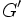# Derived subgroup not is powering-invariant

This article gives the statement, and possibly proof, of the fact that for a group, the subgroup obtained by applying a given subgroup-defining function (i.e., derived subgroup) does not always satisfy a particular subgroup property (i.e., powering-invariant subgroup)
View subgroup property satisfactions for subgroup-defining functions$|$ View subgroup property dissatisfactions for subgroup-defining functions

## Statement

It is possible to have a group$G$ such that the derived subgroup$G'$ is not a powering-invariant subgroup of$G$. Explicitly, this means that there exists a prime number$p$ such that$G$ is powered over$p$ but the derived subgroup$G'$ is not.

## Related facts

Both the facts below are strictly weaker, but it is relatively easier to construct the groups that provide examples for these, hence they may be more useful in that regard:

## Proof

Taking the free$\{ p \}$-powered group on a set of size two or more works.